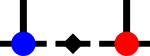SyTenlat Directory ReferenceDirectory dependency graph for lat:

## Files

file  syten-fT-mps-spin.cpp
A finite temperature spin chain lattice with U(1) symmetry.

file  syten-ipeps2-1x1-spin.py
Create an iPEPSv2 state and lattice with an effective 1x1 unit cell by placing appropriate identities and one-dimensional local spaces on the three other sites of the actual 2x2 unit cell.

file  syten-mps-boson-spinless-nil.cpp
Creates a model of spinless bosons with no symmetries.

file  syten-mps-boson-spinless-u1.cpp
Creates a U(1)-symmetric model of spinless bosons.

file  syten-mps-fermi-hubbard-qc.cpp
Quantum chemistry model with two- and four-operator terms.

file  syten-mps-fermi-hubbard-u1su2.cpp
Creates a U(1)_N×SU(2)_S Fermi-Hubbard model.

file  syten-mps-fermi-hubbard-u1u1.cpp
Creates a U(1)×U(1) Fermi-Hubbard model.

file  syten-mps-klm.cpp
Creates a $$\mathrm{U}(1)_N \times \mathrm{SU}(2)_S$$ (charge × spin)-symmetric Kondo Lattice Model.

file  syten-mps-spin-disorder-avg.py
Constructs a square MPS lattice with periodic boundary conditions and some kind of disorder.

file  syten-mps-spin-su3.cpp
Creates a SU(3) symmetric spin model.

file  syten-mps-ssh-fermi-bose.cpp
Creates a model of bosonic and fermionic sites, with no symmetries (bosons) and u1u1 symmetries (fermions)

file  syten-sql-mps-fermi-hubbard-kns.cpp
Creates a $${\mathbb{Z}_W} \times \mathrm{U}(1) \times \mathrm{SU}(2)$$ (momentum × charge × spin)-symmetric or a $${\mathbb{Z}_W} \times \mathrm{U}(1) \times \mathrm{U}(1)$$ (momentum × charge × z-spin)-symmetric 2D real/momentum space Fermi-Hubbard model.

file  syten-sql-mps-fermi-hubbard.cpp
Creates a square Fermi-Hubbard $$\mathrm{SU}(2)_{\textrm{Spin}} \times \mathrm{U}(1)_{\textrm{Charge}}$$ lattice.

file  syten-sql-mps-spin.cpp
Creates a square spin lattice.

file  syten-sql-mps-tJ.cpp
Creates a square t-J lattice with $$\mathrm{U}(1)_N \times \mathrm{U}(1)_{S^z}$$ symmetry.